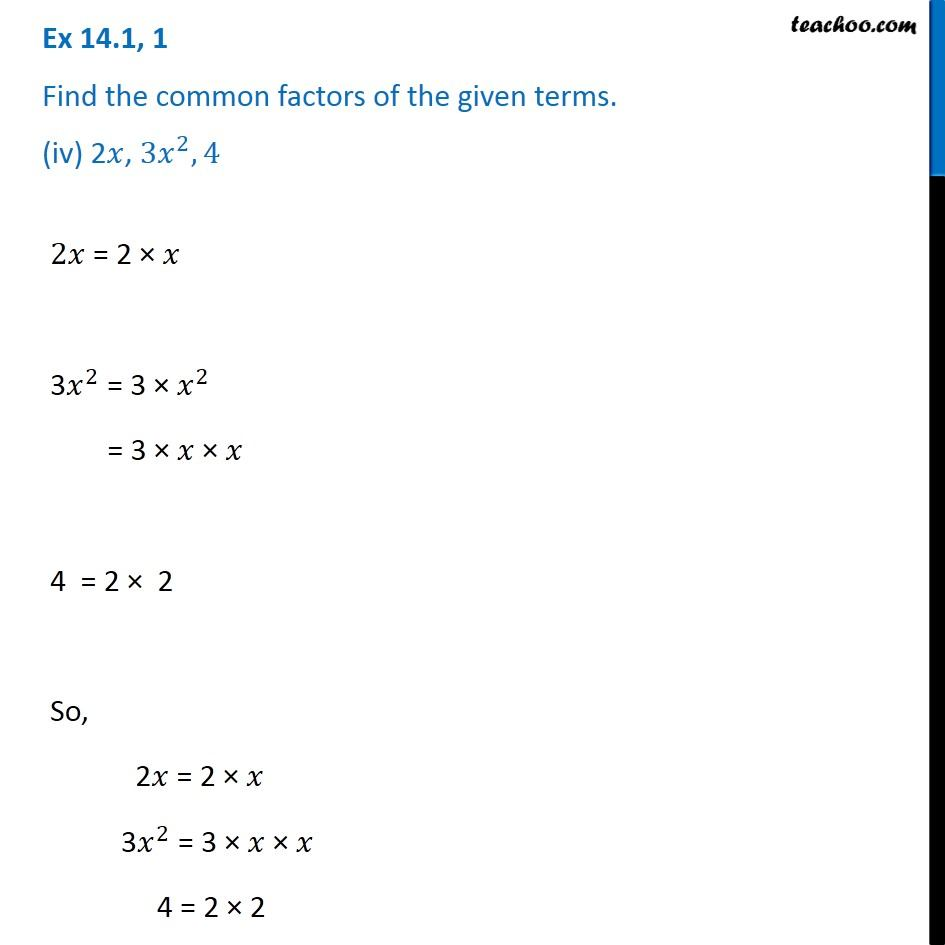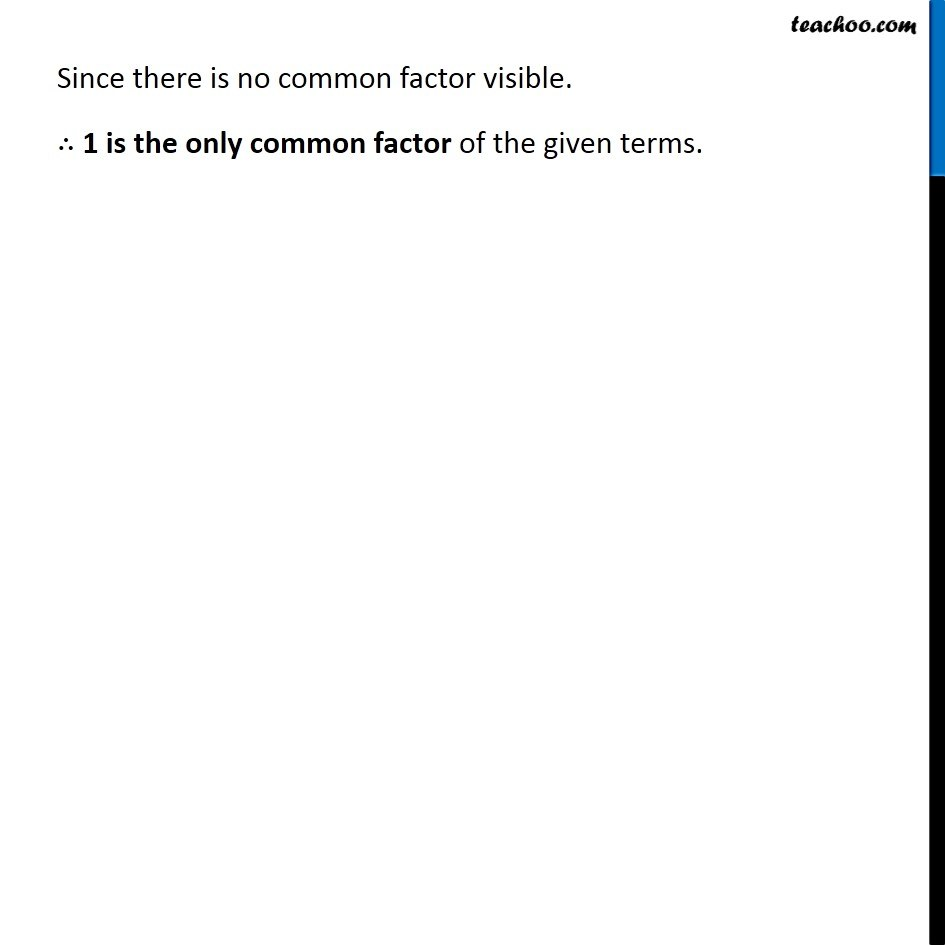Ex 14.1

Chapter 14 Class 8 Factorisation
Serial order wiseGet live Maths 1-on-1 Classs - Class 6 to 12

### Transcript

Ex 14.1, 1 Find the common factors of the given terms. (iv) 2𝑥, 〖3𝑥〗^2,4 2𝑥 = 2 × 𝑥 3𝑥^2 = 3 × 𝑥^2 = 3 × 𝑥 × 𝑥 4 = 2 × 2 So, 2𝑥 = 2 × 𝑥 3𝑥^2 = 3 × 𝑥 × 𝑥 4 = 2 × 2 Since there is no common factor visible. ∴ 1 is the only common factor of the given terms.